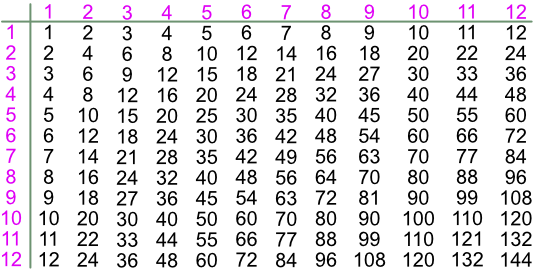Multiplication Tables

# Multiplication Tables

Basic

Multiplication tables should be recalled from memory, up to 12 x 12.

A Multiplication can be calculated either way round, 4 x 3 is the same as 3 x 4.

In descriptions, a multiplication may often be indicated by other words, such as 8 lots of 8, or contains.## Examples

1. What is 5 x 6?